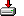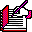#sumpuzzle.sas```/*
* Richard A DeVenezia
* http://www.devenezia.com
* Coded May 5, 1997
* Updated Dec 15, 2003 (continue instead of goto)
*/

/* Problem from posting in sci.math */

/*   A B C
* + D E F
*   -----
*   G H I
*
* where A - I are digits 1 - 9, no repeats
*/

options nosource nomprint;
options   source   mprint;
```
```%macro SumPuzzle;

%do i = 1 %to 9;
%let v&i = %sysfunc(byte(%eval(64+&i)));
%end;

data _null_;

nn = 0;
nnn = 0;
i0=0;

%do i = 1 %to 9;
do &&v&i = 1 to 9;
%do j = 1 %to %eval(&i-1);
if &&v&i = &&v&j then continue;
%end;
%end;

x = 100 * A + 10 * B + C ;
y = 100 * D + 10 * E + F ;
z = 100 * G + 10 * H + I ;

nnn + 1;

if x + y = z then do;
nn + 1;
put nn 4. +3 x '+ ' y '= ' z;
end;

%do i = 9 %to 1 %by -1;
end;
%end;

put nnn 'combinations checked';
run;
%mend;

```
```%macro SumPuzzle2;
data _null_;

do A = 1 to 4;

do B = 1 to 9;

if B=A then continue;

do C = 1 to 9;

if C=B then continue;
if C=A then continue;

do D = A+1 to 9-A;

if D=C then continue;
if D=B then continue;
if D=A then continue;

do E = 1 to 9;

if E=D then continue;
if E=C then continue;
if E=B then continue;
if E=A then continue;

do F = 1 to 9 ;

if F=E then continue;
if F=D then continue;
if F=C then continue;
if F=B then continue;
if F=A then continue;

sum = (A+D)*100+(B+E)*10+(C+F);

nnn+1;

if sum > 999 then continue;

G = int (sum/100);
I = mod (sum,10);
H = mod ((sum-I)/10,10);

if I = 0 then continue;
if H = 0 then continue;

if G=A then continue;
if G=B then continue;
if G=C then continue;
if G=D then continue;
if G=E then continue;
if G=F then continue;

if H=A then continue;
if H=B then continue;
if H=C then continue;
if H=D then continue;
if H=E then continue;
if H=F then continue;
if H=G then continue;

if I=A then continue;
if I=B then continue;
if I=C then continue;
if I=D then continue;
if I=E then continue;
if I=F then continue;
if I=G then continue;
if I=H then continue;

nn+1;
put nn 4.
' ' A +(-1) B +(-1) C '+ ' D +(-1) E +(-1) F '= ' G +(-1) H +(-1) I
'= ' D +(-1) E +(-1) F '+ ' A +(-1) B +(-1) C;

end;
end;
end;
end;
end;
end;

put nnn 'sums checked';
run;
%mend;

%SumPuzzle;
%SumPuzzle2;```

Ted Armitage sent me a great logical analysis

```Hi Richard,

Very interesting, but it probably doesn't need a computer.

Each column must have an even number of odd digits, unless it receives a
carry. There are 5 odd digits.

So there must be exactly one column which produces a carry. The column to
its left will receive the carry and the other column can come either first
or last.

Each solution will generate 16 solutions by swapping digits vertically or by
moving the column that neither gives nor receives a carry.

The column which receives the carry will contain either 1 or 3 odd digits.
The other columns contain either 0 or 2 odd digits.

With this information you can estimate an upper bound on the work remaining:

The 3 odd digits in a column can be 135 (meaning 1+3+carry=5), 157, 179, or
359. The other 2 odd digits go in one column, with 3 possibilities: both
above the line, or either one below the line. This fixes the third digit in
that column and the 3 remaining digits go in the only other column. Thus
there are only 4x3 cases to be considered.

If only 1 odd digit is in the column receiving the carry then  124, 146,
168, 326, 348, 528 are the valid combinations. Next, for simplicity, work
out the column which does not produce a carry. There are 2 choices left for
the even digit. There are 6 ways to choose the 2 odd digits from the
remaining 4. This gives 6x2x6 cases.

So the total cases to consider are 12+72 = 84, most of which are likely to
be invalid, which is not too bad.

Ted
```